# Fraction IX Least Common Multiple Least Common Denominator

• Slides: 41Fraction IX Least Common Multiple Least Common Denominator By Monica Yuskaitis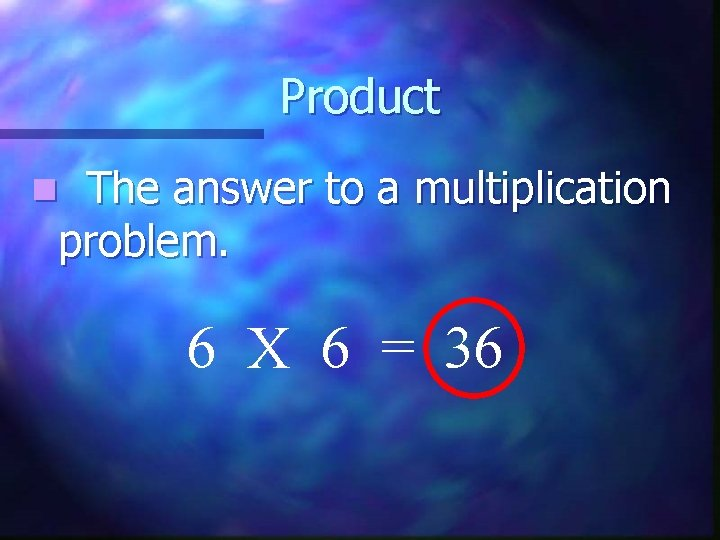Product The answer to a multiplication problem. n 6 X 6 = 36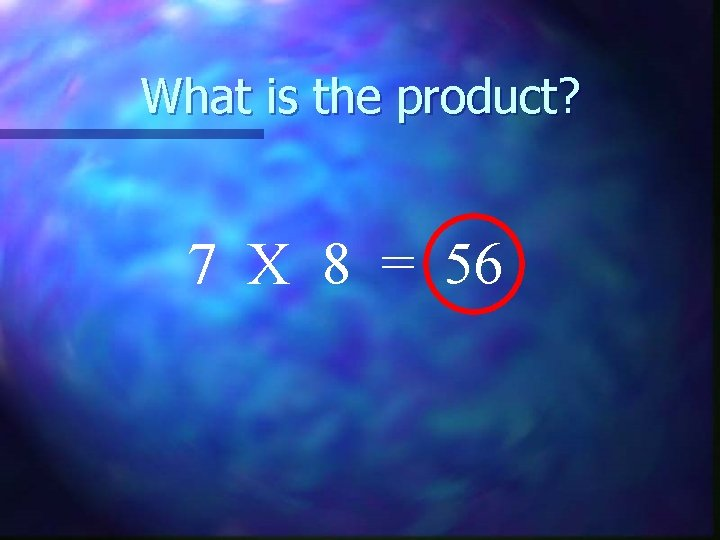What is the product? 7 X 8 = 56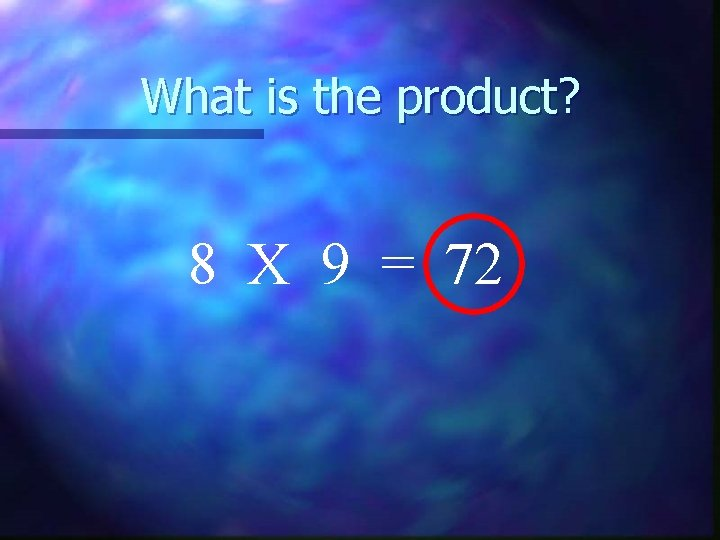What is the product? 8 X 9 = 72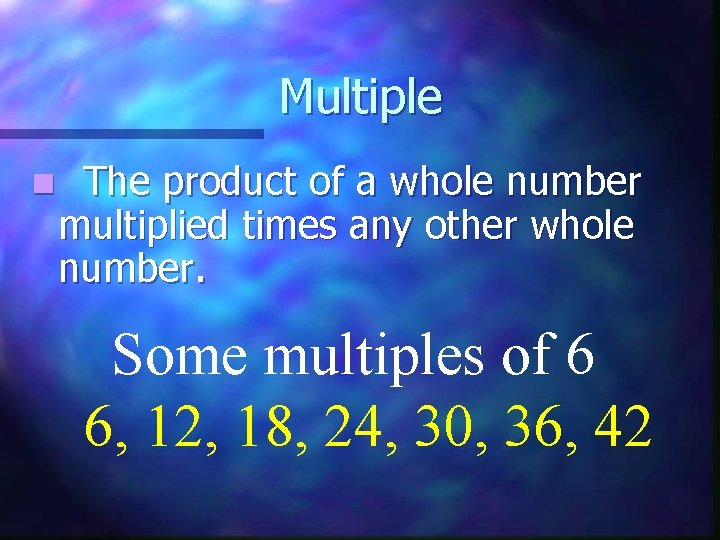Multiple n The product of a whole number multiplied times any other whole number. Some multiples of 6 6, 12, 18, 24, 30, 36, 42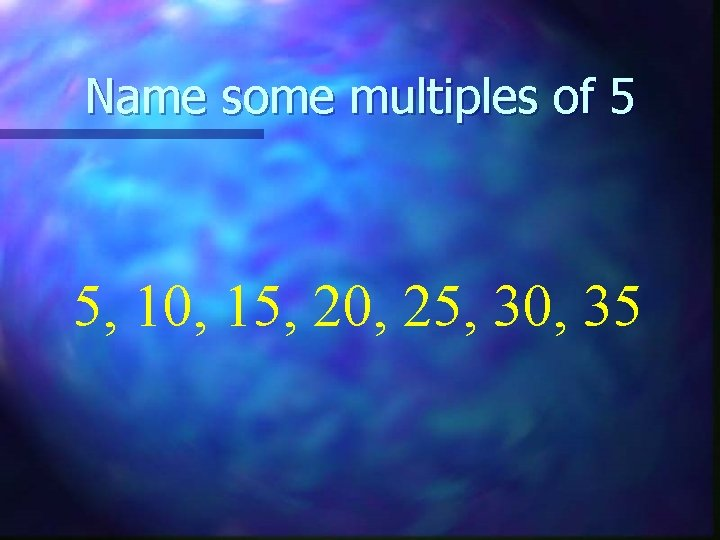Name some multiples of 5 5, 10, 15, 20, 25, 30, 35Name some multiples of 7 7, 14, 21, 28, 35, 42, 49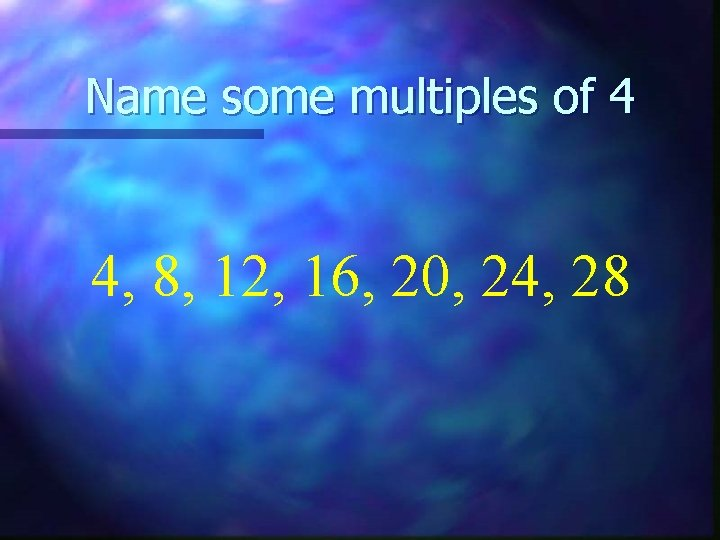Name some multiples of 4 4, 8, 12, 16, 20, 24, 28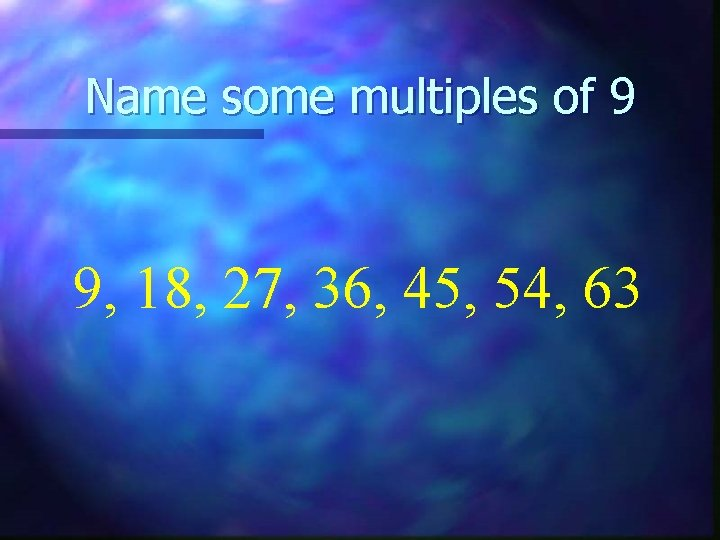Name some multiples of 9 9, 18, 27, 36, 45, 54, 63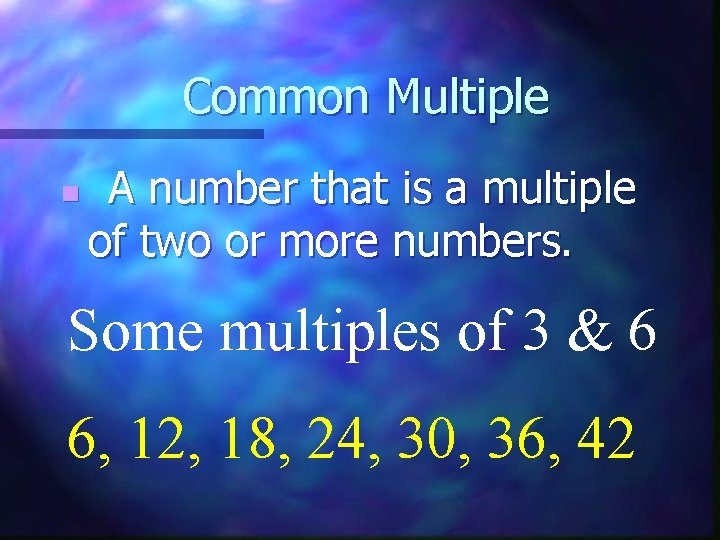Common Multiple n A number that is a multiple of two or more numbers. Some multiples of 3 & 6 6, 12, 18, 24, 30, 36, 42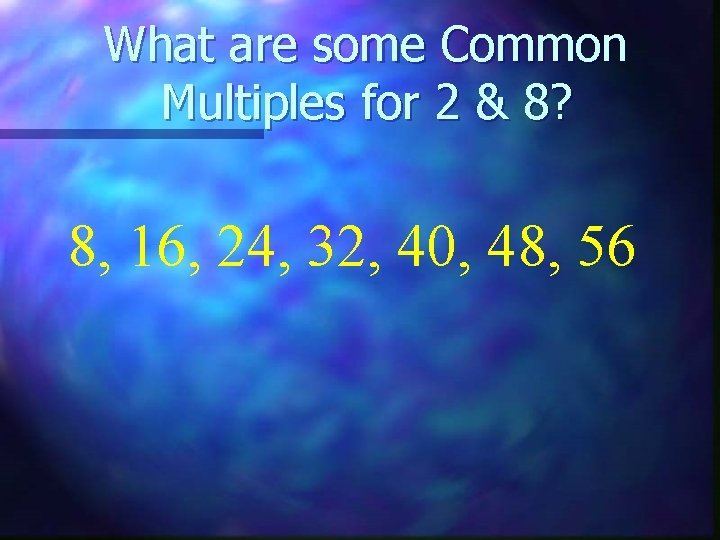What are some Common Multiples for 2 & 8? 8, 16, 24, 32, 40, 48, 56What are some Common Multiples for 4 & 6? 12, 24, 36, 48, 56, 60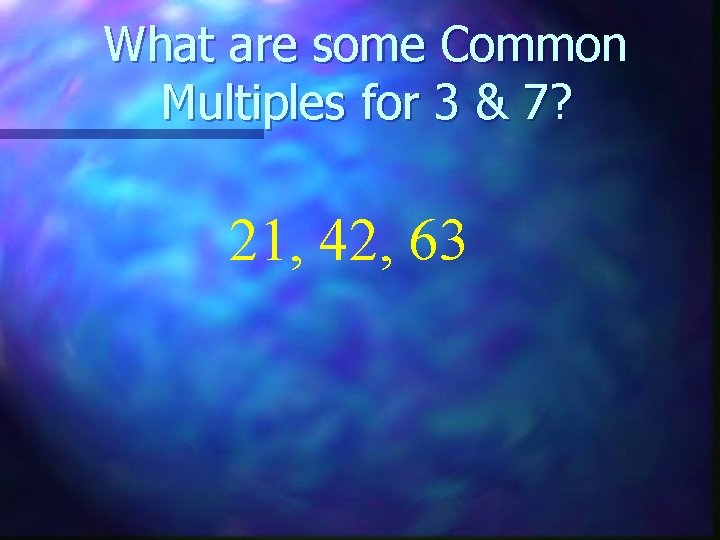What are some Common Multiples for 3 & 7? 21, 42, 63What are some Common Multiples for 5 & 10? 10, 20, 30, 40, 50, 60Least Common Multiple The smallest common multiple of a set of two or more numbers. n 5 = 5, 10, 15, 20, 25, 30 6 = 6, 12, 18, 24, 30, 36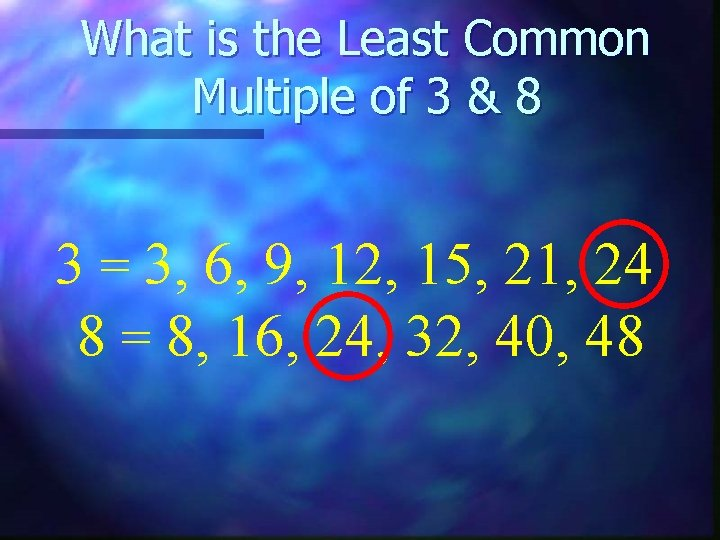What is the Least Common Multiple of 3 & 8 3 = 3, 6, 9, 12, 15, 21, 24 8 = 8, 16, 24, 32, 40, 48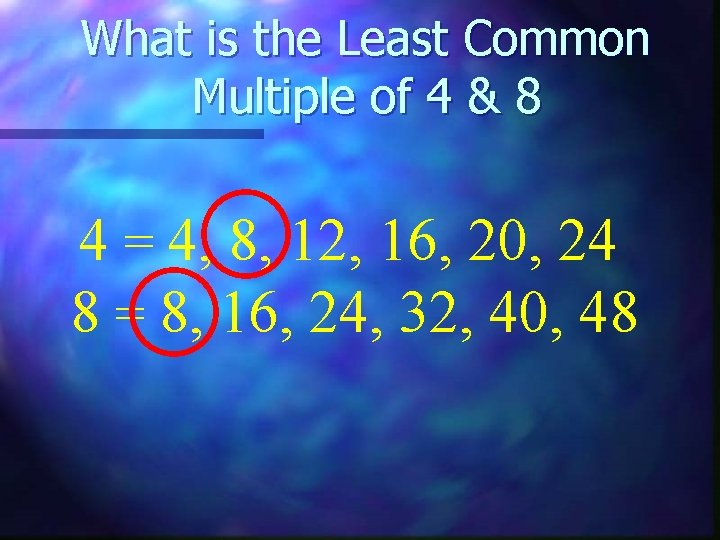What is the Least Common Multiple of 4 & 8 4 = 4, 8, 12, 16, 20, 24 8 = 8, 16, 24, 32, 40, 48What is the Least Common Multiple of 6 & 8 6 = 6, 12, 18, 24, 30, 36 8 = 8, 16, 24, 32, 40, 48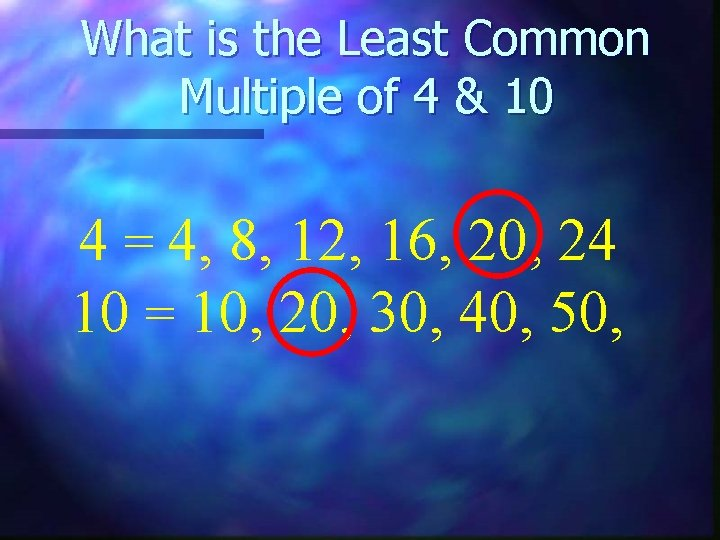What is the Least Common Multiple of 4 & 10 4 = 4, 8, 12, 16, 20, 24 10 = 10, 20, 30, 40, 50,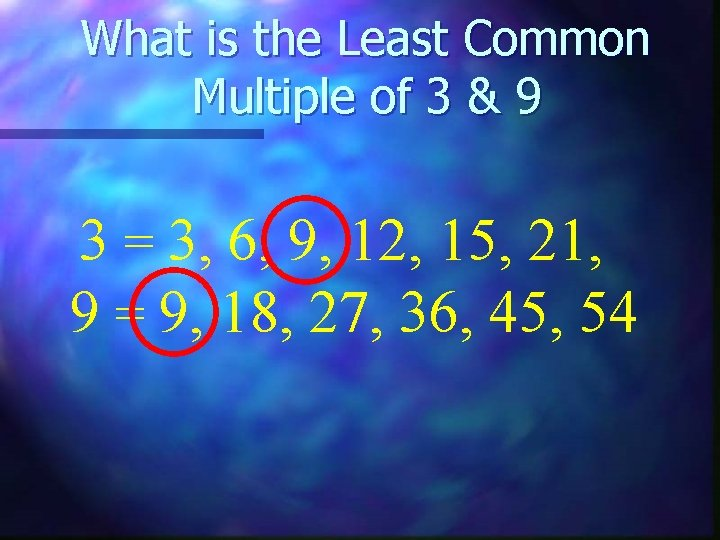What is the Least Common Multiple of 3 & 9 3 = 3, 6, 9, 12, 15, 21, 9 = 9, 18, 27, 36, 45, 54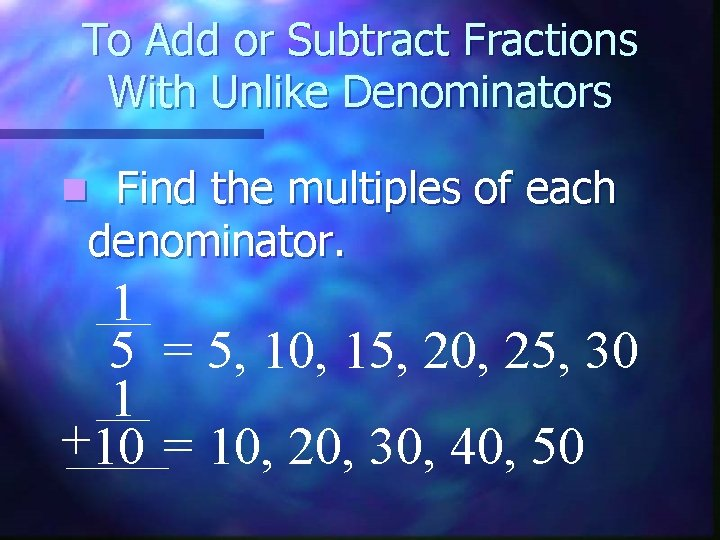To Add or Subtract Fractions With Unlike Denominators Find the multiples of each denominator. n 1 5 = 5, 10, 15, 20, 25, 30 1 +10 = 10, 20, 30, 40, 50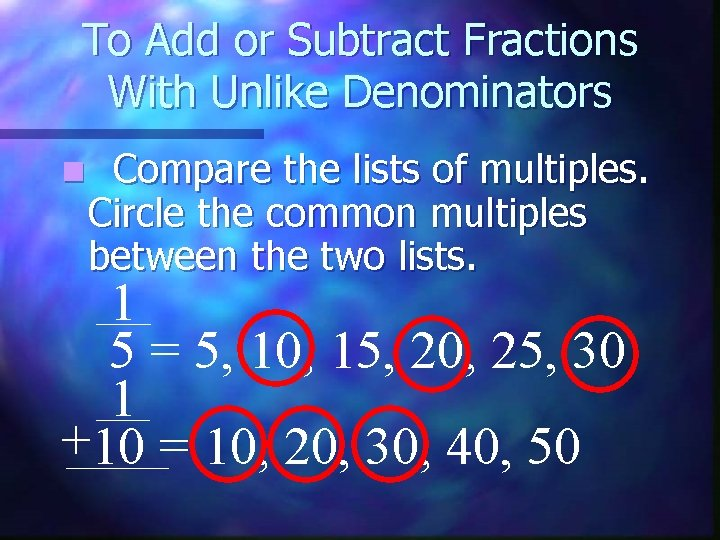To Add or Subtract Fractions With Unlike Denominators n Compare the lists of multiples. Circle the common multiples between the two lists. 1 5 = 5, 10, 15, 20, 25, 30 1 +10 = 10, 20, 30, 40, 50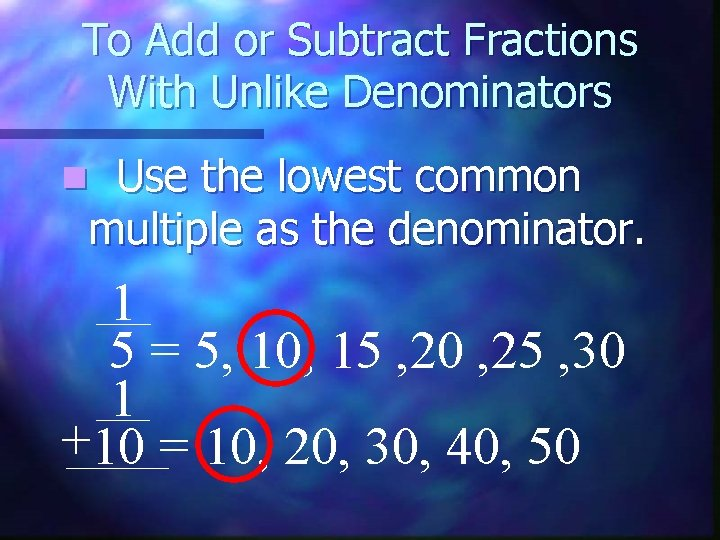To Add or Subtract Fractions With Unlike Denominators Use the lowest common multiple as the denominator. n 1 5 = 5, 10, 15 , 20 , 25 , 30 1 +10 = 10, 20, 30, 40, 50To Add or Subtract Fractions With Unlike Denominators This number is also called the least common denominator. n 1 5 = 5, 10, 15 , 20 , 25 , 30 1 +10 = 10, 20, 30, 40, 50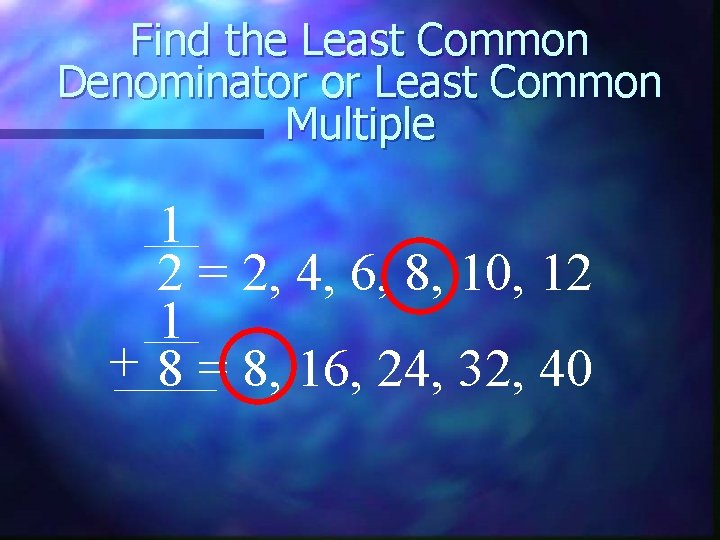Find the Least Common Denominator or Least Common Multiple 1 2 = 2, 4, 6, 8, 10, 12 1 + 8 = 8, 16, 24, 32, 40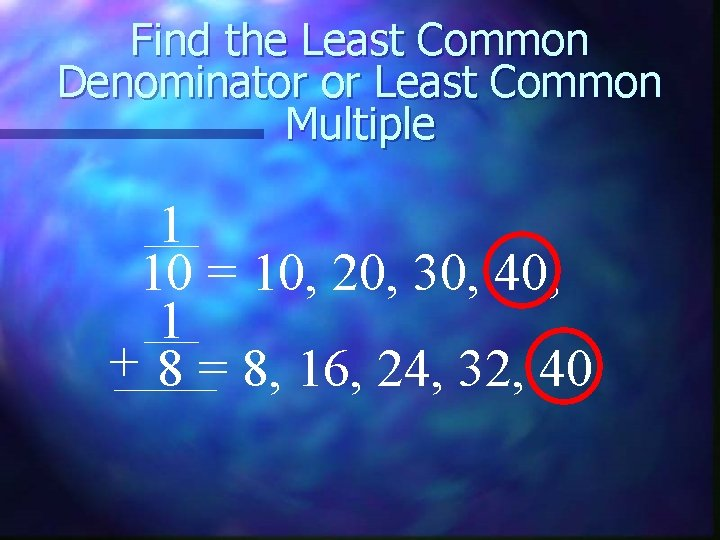Find the Least Common Denominator or Least Common Multiple 1 10 = 10, 20, 30, 40, 1 + 8 = 8, 16, 24, 32, 40Find the Least Common Denominator or Least Common Multiple 1 10 = 10, 20, 30, 40, 1 + 4 = 4, 8, 12, 16, 20,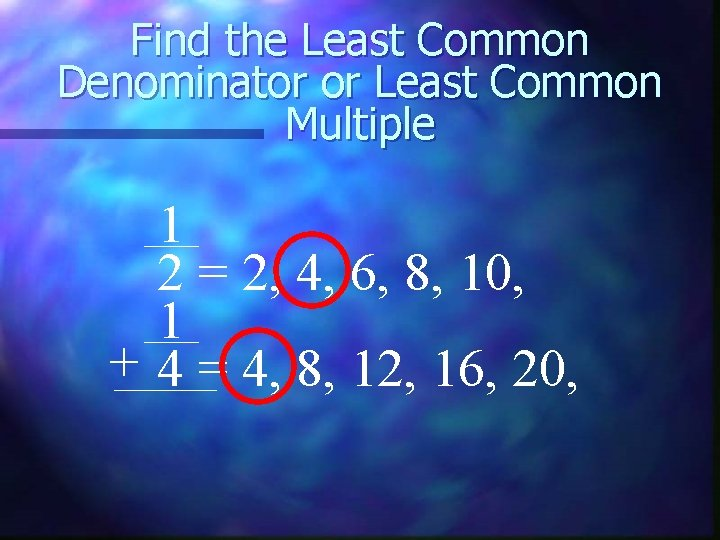Find the Least Common Denominator or Least Common Multiple 1 2 = 2, 4, 6, 8, 10, 1 + 4 = 4, 8, 12, 16, 20,Find the Least Common Denominator or Least Common Multiple 1 2 = 2, 4, 6, 8, 10, 12 1 + 12 = 12, 24, 36, 48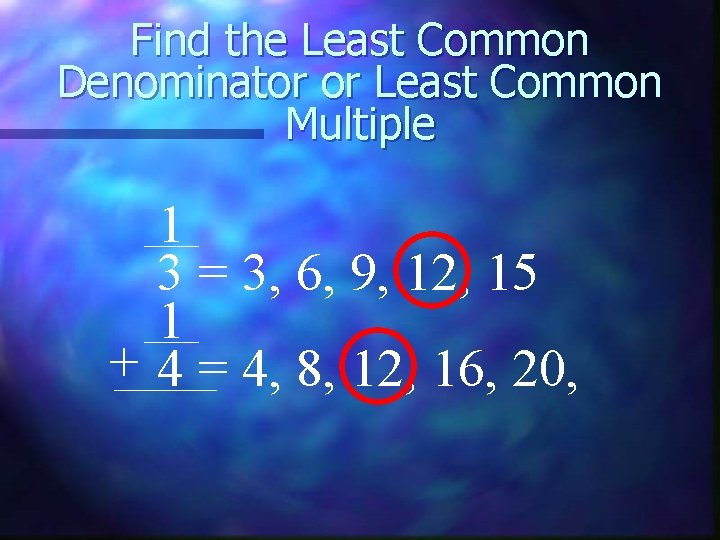Find the Least Common Denominator or Least Common Multiple 1 3 = 3, 6, 9, 12, 15 1 + 4 = 4, 8, 12, 16, 20,Find the Least Common Denominator or Least Common Multiple 1 3 = 3, 6, 9, 12, 15 1 + 5 = 5, 10, 15, 20, 25,Find the Least Common Denominator or Least Common Multiple 1 3 = 3, 6, 9, 12, 15 1 + 9 = 9, 18, 27, 36, 45,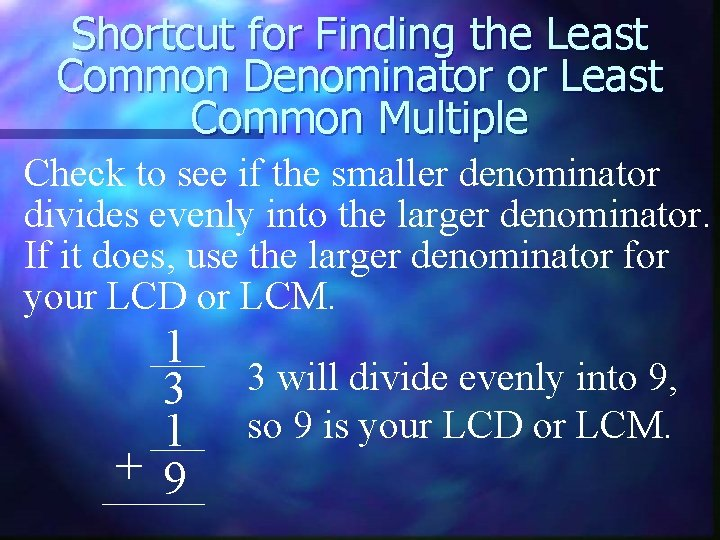Shortcut for Finding the Least Common Denominator or Least Common Multiple Check to see if the smaller denominator divides evenly into the larger denominator. If it does, use the larger denominator for your LCD or LCM. 1 3 1 + 9 3 will divide evenly into 9, so 9 is your LCD or LCM.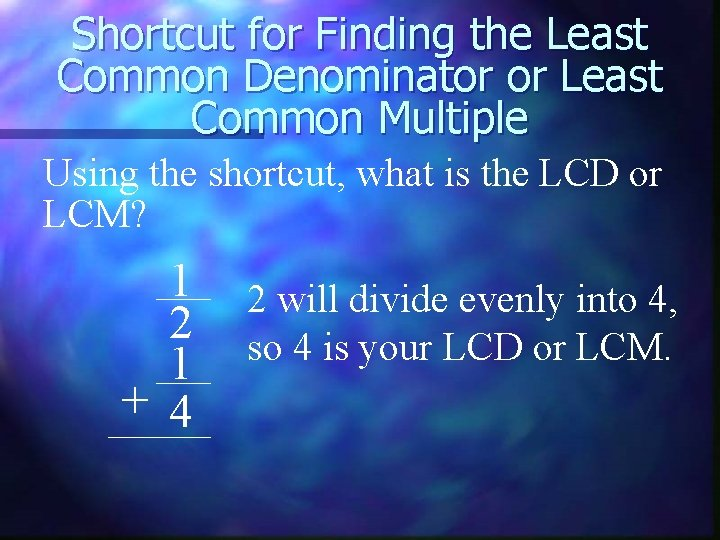Shortcut for Finding the Least Common Denominator or Least Common Multiple Using the shortcut, what is the LCD or LCM? 1 2 1 + 4 2 will divide evenly into 4, so 4 is your LCD or LCM.Shortcut for Finding the Least Common Denominator or Least Common Multiple Using the shortcut, what is the LCD or LCM? 1 2 will divide evenly into 10, 2 so 10 is your LCD or LCM. 1 + 10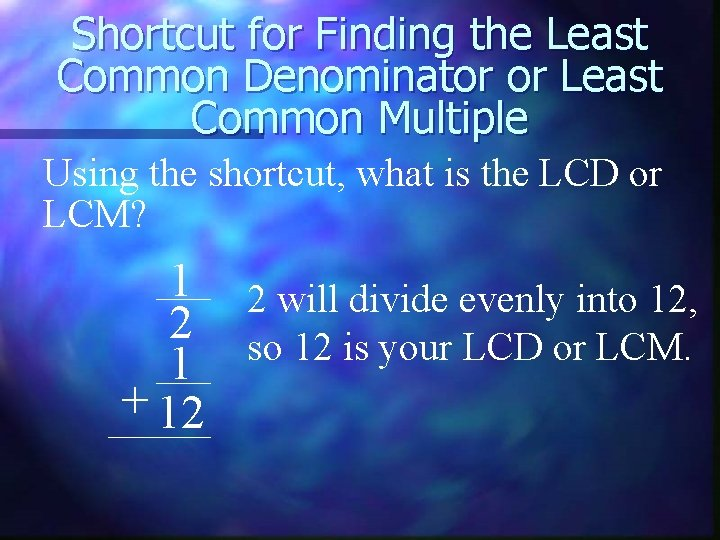Shortcut for Finding the Least Common Denominator or Least Common Multiple Using the shortcut, what is the LCD or LCM? 1 2 will divide evenly into 12, 2 so 12 is your LCD or LCM. 1 + 12Shortcut for Finding the Least Common Denominator or Least Common Multiple Using the shortcut, what is the LCD or LCM? 1 4 1 + 8 4 will divide evenly into 8, so 8 is your LCD or LCM.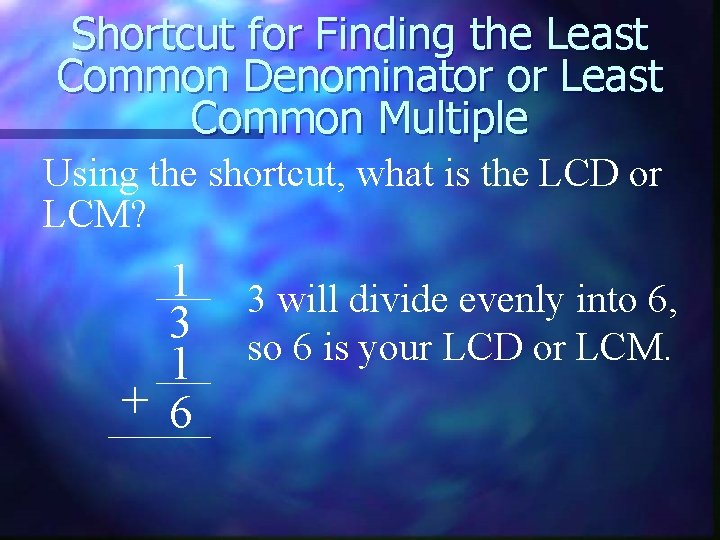Shortcut for Finding the Least Common Denominator or Least Common Multiple Using the shortcut, what is the LCD or LCM? 1 3 1 + 6 3 will divide evenly into 6, so 6 is your LCD or LCM.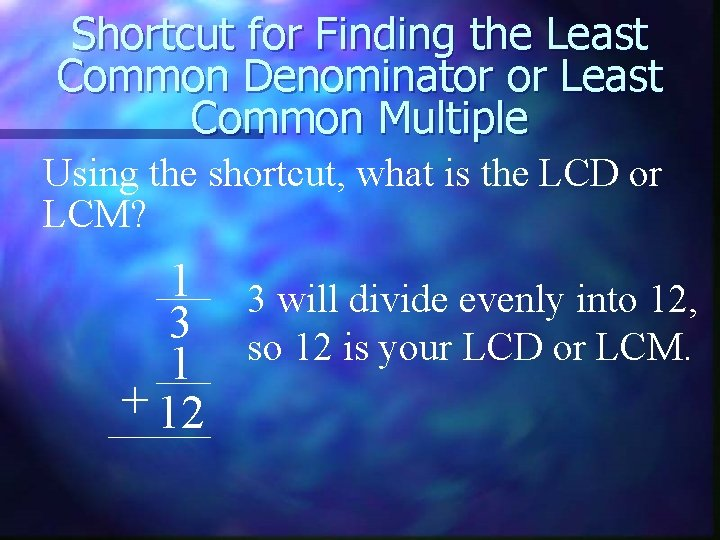Shortcut for Finding the Least Common Denominator or Least Common Multiple Using the shortcut, what is the LCD or LCM? 1 3 will divide evenly into 12, 3 so 12 is your LCD or LCM. 1 + 12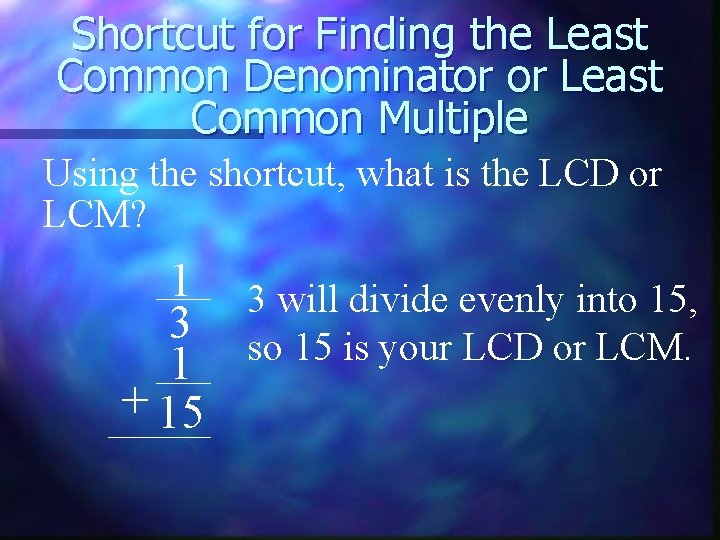Shortcut for Finding the Least Common Denominator or Least Common Multiple Using the shortcut, what is the LCD or LCM? 1 3 will divide evenly into 15, 3 so 15 is your LCD or LCM. 1 + 15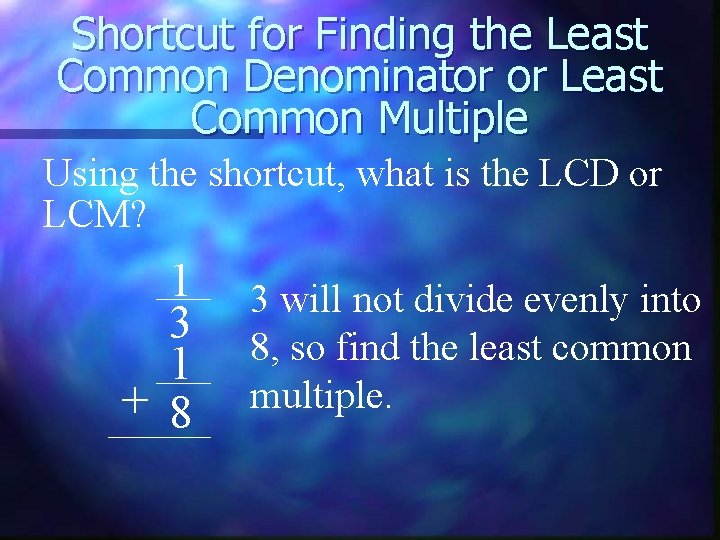Shortcut for Finding the Least Common Denominator or Least Common Multiple Using the shortcut, what is the LCD or LCM? 1 3 1 + 8 3 will not divide evenly into 8, so find the least common multiple.